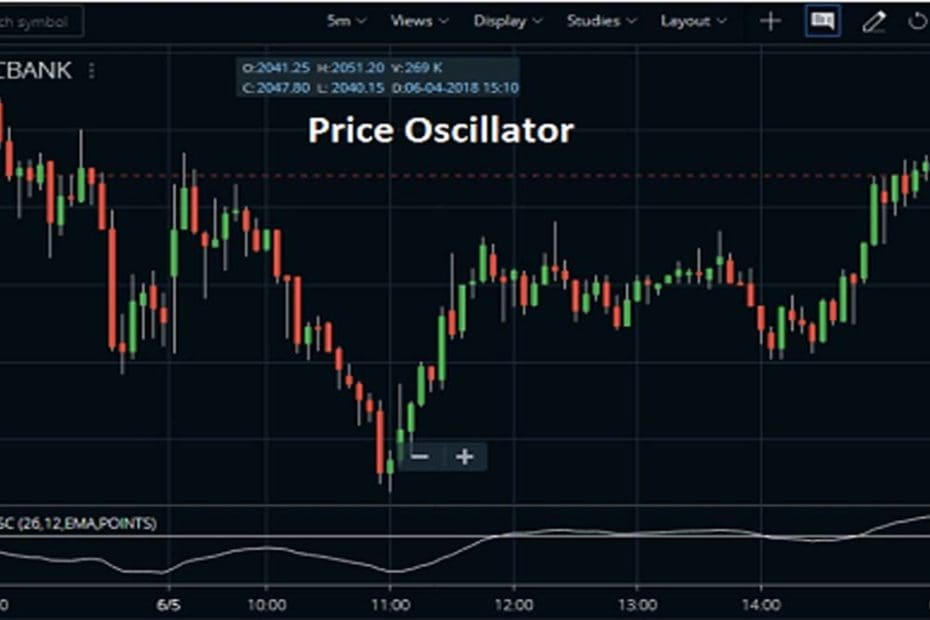Home » Blog » Trading » Technical Indicators » Price Oscillator Indicator (PPO) Calculation, Trading Tactics

# Price Oscillator Indicator (PPO) Calculation, Trading Tactics

• Technical IndicatorsThe Price Oscillator Indicator, also known as the percentage price oscillator is a technical analysis tool, used for measuring momentum. This indicator mainly suggests the overbought and oversold areas, confirm bullish, bearish price moves. This article contains its easy calculation method, trading tactics.

## What is Price Oscillator or Percentage Price Oscillator?

The Price Oscillator shows the relationship between two moving averages in percentage terms. That’s why it is often termed as the Percentage price oscillator. Generally, traders use this PPO or Percentage Price Oscillator to compare the asset performance, volatility, divergence, trading signals and, lots more.

### Key Notes of the Price Oscillator

• The PPO or Percentage Price Oscillator contains 2 lines, the PPO line, and the signal line.
• The signal line is an EMA of the PPO. Hence, it moves slower than the PPO.
• Sometimes, traders prefer the PPO because readings are comparable between assets with various prices, whereas MACD readings are not comparable.

## What is the Relationship between PPO and MACD?

The PPO is almost identical to the moving average convergence divergence (MACD) technical indicator. They both are the momentum oscillator, measure the difference between the two moving averages. But still, there are differences between the two, stated below:

### Difference between the Two, PPO & MACD

• Here, PPO measures the percentage difference between the two EMAs, while the MACD measures the absolute (dollar) difference.
• MACD(12,26,9) computed the absolute difference between the 12-day and 26-day exponential moving averages (EMA). On the opposite side, PPO(12,26,9) takes MACD one step further by showing the percentage difference between these two MA.

## How to Calculate Price Oscillator?

PPO Line is taking as a result of long-term EMA and subtracting it from a shorter-term EMA. Then the result is divided by the longer-term EMA and then multiplied by 100. The used values are 26 days EMA (for the longer-term EMA) and 12 days EMA (for the shorter term EMA) of this PPO line which is trader can choose this value.

Here, are the detailed explanations of the above formula.

1. First, calculate the 12-period EMA of the asset’s price.
2. Then, calculate the 26-period EMA of the asset’s price.
3. After that, apply these to the PPO formula to get the current PPO value.
4. Now, once there are at least nine PPO values, originate the signal line by calculating the nine-period EMA of the PPO.
5. To formulate a histogram reading, subtract the current PPO value from the current signal line value. So, the histogram is an optional visual representation of the distance between these two lines.

## How to Set up the PPO Indicator on your Charts?

To set up the PPO indicator, you need to open the terminal first. Here, I’ve taken Zerodha Kite and Upstox Pro to set the examples.

### Set up the PPO Indicator on Zerodha Kite

To attach the Percentage Price Oscillator (PPO) indicator, traders can find under the STUDIES section in the Zerodha Kite browser app and it is also available in the Kite mobile App. The Field is close or you can also choose from OHLC. We can also set the field Short Cycle at 12 and we can these values high or low. The Moving Average Type is simple/exponential etc and we also change this. Please check the image below to understand how we attached the PPO indicator in HDFC Bank share price chart. You can attach the PPO indicator on to any charts likes daily, weekly, monthly or intraday.

### Set up the PPO Indicator on Upstox Pro

The setup process in Upstox pro is almost similar to Zerodha Kite. You need to log in to your account first, then open the chart and indicator section. There put down the indicator name and click on the Apply option.

## Top Trading Tactics of Price Oscillator Indicator

• When the PPO is above zero that helps indicate an uptrend, as the short-term EMA is above the longer-term EMA.
• When the PPO is below zero, the short-term average is below the longer-term average, which helps indicate a downtrend.
• Here, one more thing traders should consider that if the price goes at or above 2.5, it is overbought. Oppositely, when the price goes at or below (-2.5), it is oversold.
• Traders use the PPO crossing the signal line as a trade signal. Once it crosses above from below, that is a buy, when the prices cross below from above that is a sell.

In order to avoid noise, here I add 100 MA (Moving average). Once the price crosses the MA above buy at the crossing point and sells at the down line. In this way, you can easily ignore the extra noise.

### Conclusion :

The Price Oscillator Indicator or PPO generates the same signals of the MACD. It also provides an added dimension as a percentage version of MACD. The PPO levels of the Nifty 50 (price ~10700) can be compared against the PPO levels of Sun Pharma (price ~490) because of the PPO “levels” the trading field. Also, PPO levels in one security can be compared over extended periods of time, even if the price has doubled or tripled. This is not the case for the MACD. The advantage of this Percentage Price Oscillator (PPO) is this technical indicator is still not the best oscillator to identify overbought or oversold conditions because movements are unlimited.